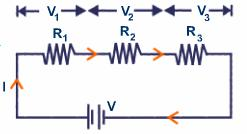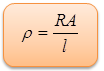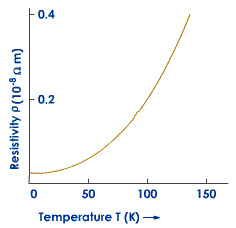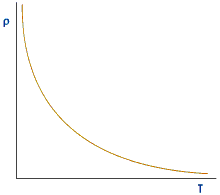Click to Chat

1800-1023-196

+91-120-4616500

CART 0

• 0

MY CART (5)

Use Coupon: CART20 and get 20% off on all online Study Material

ITEM
DETAILS
MRP
DISCOUNT
FINAL PRICE
Total Price: Rs.

There are no items in this cart.
Continue Shopping• Complete Physics Course - Class 11
• OFFERED PRICE: Rs. 2,968
• View Details

Resistance and Resistivity

Table of Content

Resistance

Concept of Resistance

Resistance in Series

Resistance in Parallel

Variation of Resistance with Temperature

Resistivity

Variation of Resistivity with Temperature

Insulators

Related Resources

ResistanceIf ‘V’ be the potential difference between the two terminals of a conductor and ‘i’ be the current through it, then,

V/i = Constant = R

‘R’ is called the resistance of the material.An increase in the value of R results in a decrease in the value of ‘i’.

Quantitative definition: Resistance is the opposition offered by the conductor to the flow of electricity through it.

Quantitative definition: Resistance of a conductor is defined as the ratio between potential differences between the two ends of the conductor to the current flowing through it.

If i = 1, R = V.

Resistance of a conductor can also be defined as the difference of potential across the two ends of the conductor required to pass a unit electric current through it.

Concept of Resistance

Every conductor contains a large number of free electrons. When a difference of potential is applied between the two ends of the conductor, an electric field is set up inside the material of the conductor. A free electron (being a negatively charged particle) experiences a force, due to this field, which accelerates it from higher to lower potential side. After acquiring some velocity it suffers collision with other free electrons of the material and loses the acquired energy. It, again, is accelerate and goes through the above process repeatedly. Thus, motion of the electron cannot be termed as free. It experiences resistance forward motion. This resistance is termed as electrical resistance.

Units of R:-

(a) In S.I:- 1 ohm = 1 volt/1 ampere

Resistance of a conductor is said to be ‘ohm’ if a current of 1 ampere flows through it for a potential difference of 1 volt across its ends.

(b) In C.G.S system:-

1 statohm = 1 statvolt/1 statamp

Resistance of a conductor is said to be 1 statohm if a current of 1 statamp flows through it for a potential difference of 1 statvolt across its ends.

1 abohm = 1 abvolt/1 abampere

Resistance of a conductor is said to be 1 abohm if a current of 1 abampere flows through it for a potential difference of 1 abvolt across its ends.

(c) Relation between ohm and statohm:-

1 ohm = 1 volt/1 ampere

= [(1/300) statvolt] / [(3109) statamp]

Thus, 1 ohm = (1/9×1011) statohm

(d) Relation between ohm and abohm:-

1 ohm = 1 volt/1 ampere

1 ohm = [108 abvolt]/ [(1/10) abamp]

Thus, 1 ohm = 109abohm

Resistance in SeriesThe resistances are said to be connected in series if same current flows through all of them. Consider resistances R1, R2, and R3 connected in series with each other as shown in figure. Let a current ‘i’ flow through all of them. If V1, V2 and V3 are difference of potentials

V1 = iR1, V2 = iR2 and V3 = iR3 across each resistance, R is the resistance of combination, total potential difference “V’ across whole of the combination is

V = iR

Since V = V1+V2 + V3

So, iR = iR1+iR2+iR3

or iR = i (R1+R2+R3)

or R = R1 + R2 + R3

Thus, if a number of resistances are connected in series with each other, the net resistance of the combination is equal to the sum of their individual resistances.

Resistance in ParallelResistances are said to be connected in parallel if different currents flow through them and get added afterwards. Consider a number of resistances R1, R2, and R3 connected parallel to each other. A current ‘i’ is divided into three parts and flows through each of these resistances as shown in figure. If ‘V’ is the difference of potential across the combination, then

V = i1R1 = i2R2 = i3R3

or i1 = V/R1, i2 = V/R2 , i3 = V/R3

If R is the resistance of the combination, then

i = V/R

Since i = i1 +i2+ i3

So, V/R = V/R1 +V/R2 + V/R3

or V/R = V (1/R1 +1/R2 +1/R3)

1/R = 1/R1 +1/R2 +1/R3

Thus , if a number of resistances are connected in parallel, the reciprocal of the resistance of the combination is equal to the sum of the reciprocals of their individual resistances.

Variation of Resistance with Temperature

Resistance of a conductor varies with temperature. If ‘R0’ and ‘Rt’ are its resistance at 0º C and tº C respectively, then

Rt = R0 (1+αt)

or, Rt = R0+R0αt

R0αt = Rt – R0

So,α = Rt – R0/R0t

Where,‘α’ is known as temperature coefficient of resistance.

Temperature coefficient of resistance is defined as change in resistance of the conductor per unit resistance per degree centigrade rise of temperature.

Its S.I. unit is C-1.

(a) For all metals and most of alloys ‘α’ is positive, i.e., their resistance increases with an increase in their temperature.

As a substance is heated, its electrons start vibrating more vigorously and suffer collisions more frequently. A larger number of collisions means more resistance. Hence, resistance increases with the rise in temperature.

Value of ‘α’ is greater for metals and smaller for alloys. Therefore metals show more change in resistance, as compared to alloys, when they are heated. This is the basic reason why alloys are used in resistance boxes and metals are used in the construction of resistance thermometers.

(b) Substances like carbon and semi-conductors possess negative value of ‘α’. Their resistance decreases with a rise in temperature.

Watch this Video for more reference

Resistivity

If ‘V’ be the potential difference between the two terminals of a conductor and ‘i’ be the current through it, then

V/i = Constant = R

‘R’ is called the resistance of the material.

i = V/R

An increase in the value of R results in a decrease in the value of ‘i’.

Thus, resistance is the opposition offered by the conductor to the flow of electricity through it.

From Ohm’s law

R = (ml/nAe2) (1/)      …... (1)

As is clear from equation (1), resistance ‘R’ of a conductor depend on the following factors:

(a) Length ‘l’ of the conductor:

R ∝ l

That is, resistance of conductor varies directly as its length.

As potential difference is applied across the two ends, free electrons move from the end at lower potential to the end at a higher potential. In this process they collide against each other and undergo retardation. A greater length of conductor results in greater number of collisions, thereby producing greater retardation and hence greater resistance.

(b) Area of cross-section ‘A’:

R∝1/A

Resistance of a conductor varies inversely as its area of cross-section.

For a conductor having greater area of cross-section, more free electrons cross that section of conductor in one second, thereby giving a large current. A large current means a lesser resistance.

Combining the two factors together, we get

R∝ l/A

or, R = l/A

Here ‘’ is the resistivity or the specific resistance of the material.

If l = 1, A = 1 then R = .

Thus, the resistivity of material is numerically equal to the resistance of a conductor made up of the material of unit length and unit area of cross-section.

Alternatively, it is also numerically equal to the resistance in between the two opposite faces of a unit cube made up of that material.So the unit will be,

ohm m2/m = ohm meter

Units of resistivity are ‘ohm meter’.

Variation of Resistivity with TemperatureThe resistivity of a metallic conductor nearly always increases with increasing temperature. As temperature increases, the ions of the conductor vibrate with greater amplitude, making it more likely that a moving electron will collide with an ion. This impedes the drift of electrons and hence the current. Over a small temperature range, the resistivity of a metal can be represented by a linear relation

where ro is the resistivity at a reference temperature To and r (T) is the resistivity at temperature T. a is called the temperature coefficient of resistivity and has dimensions of (oC)-1.

However, the temperature dependence of r at low temperatures is non-linear as shown in figure given below.

InsulatorsIn case of insulators the resistivity, almost, increases exponentially with a decrease in temperature and tends to infinity as the temperature approaches 0 K. Resistivity ρ0 and ρt at 0ºC and tºC, respectively, are connected by the relation

ρt = ρ0 e-Eg/kT          …... (1)

Where ‘Eg’ is called the [positive energy of the insulator and ‘k’ is Boltzmann constant. Depending upon the value of Eg, the substance behaves like an insulator or as a semi-conductor.

For Eg < 1 eV, the value of ‘ρ’ at room temperature is not very high. Therefore the substance acts as a semiconductor. For Eg > 1 eV, the value of ρ at room temperature is high. So the substance act as an insulator.

Since number of electron per cm3 of the material varies inversely as the resistivity,

n∝1/p

Thus, from equation (1) nt = n0 e-Eg/kT

Here n0 has a value of the order of 1028 m-3.Resistivity is a constant of the material. Two wires having different lengths and thickness but made up of same material will have same resistivity.

Resistivity of conductor is much small while that of insulator is large.

Resistivity of a conductor increases with an increase in temperature.

Resistivity of an insulator increases with a decrease in temperature.

Resistivity and conductivity are inverse of each other.

Resistance and conductance are inverse of each other.

Problem (JEE Main)

A resistor of resistance R is connected to a cell of internal resistance 5. The value of R is varied from 1 to 5. The power consumed by R,

(a) increase continuously

(b) decreases continuously

(c) first decreases then increases

(d) first increases then decreases

Solution:

When external resistance R = internal resistance or 5 then power consumed by r is maximum. Therefore from the above observation we conclude that, option (a) is correct.Question 1

Resistivity of a wire depends on

(A) length

(B) material

(C) cross section area

(D) none of the above.

Question 2

When n resistances each of value r are connected in parallel, then resultant resistance is x. When these n resistances are connected in series, total resistance is

(A) nx

(B) rnx

(C) x / n

(D) n2 x.

Question 3

Resistance of a wire is r ohms. The wire is stretched to double its length, then its resistance in ohms is

(A) r / 2

(B) 4 r

(C) 2 r

(D) r / 4

Question 4

The element of electric heater is made of

(A) copper

(B) steel

(C) carbon

(D) nichrome.

Question 5

In resistors two wire side by side in opposite direction are wound in order to

?(a) give more mechanical strength.

?(b) increase the resistance.

?(c) make it more stable.

?(d) reduce the coil induction.Q.1
Q.2
Q.3
Q.4
Q.5

b

d

b

d

d

Related Resources

You might like to Ohm’s law.

For getting an idea of the type of questions asked, refer the  Previous Year Question Papers.

To read more, Buy study materials of Current Electricity comprising study notes, revision notes, video lectures, previous year solved questions etc. Also browse for more study materials on Physics here.### Course Features

• 101 Video Lectures
• Revision Notes
• Previous Year Papers
• Mind Map
• Study Planner
• NCERT Solutions
• Discussion Forum
• Test paper with Video Solution